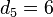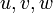# Difference between revisions of "Determination of character table of alternating group:A5"

This page describes the process used for the determination of specific information related to a particular group. The information type is character table and the group is alternating group:A5.
View all pages that describe how to determine character table of particular groups | View all specific information about alternating group:A5
This page is incomplete. At present, it only includes the determination of the degrees of irreducible representations, not the entire character table.

This page describes various ways to determine the character table of alternating group:A5. The idea is to determine as much as possible wit has little knowledge of the representation theory of these groups as we can manage, therefore making the discussion suitable for people who know only basic facts about the groups and basic facts of linear representation theory. For a detailed discussion of the character theory, see linear representation theory of alternating group:A5.

## Finding the degrees of irreducible representations using number-theoretic constraints

Final answer: The degrees of irreducible representations are 1, 3, 3, 4, 5.

The following facts are known in general:

1. Sum of squares of degrees of irreducible representations equals order of group: In this case, it tells us that the sum of the degrees of irreducible representations is 60, the order of the group.
2. Number of irreducible representations equals number of conjugacy classes: In this case, it tells us that there are 5 irreducible representations, because there are 5 conjugacy classes.
3. Degree of irreducible representation divides order of group: In this case, it tells us that the degrees of irreducible representations all divide 60, the order of the group.
4. Number of one-dimensional representations equals order of abelianization: In this case, the group is a perfect group (in fact, it is a simple non-abelian group -- see A5 is simple) so there is only one one-dimensional representation.
5. Order of inner automorphism group bounds square of degree of irreducible representation

(2) tells us that there are five irreducible representations. Denote their (positive integer) degrees, in non-decreasing order, as$d_1 \le d_2 \le \dots \le d_5$. (1) tells us that$d_1^2 + d_2^2 + d_3^2 + d_4^2 + d_5^2 = 60$. Note that$d_5 \le 7$ because$8^2 = 64 > 60$. Moreover, (3) tells us that all the degrees must divide 60, this eliminates the case$d_5 = 7$. There exists a trivial representation, so$d_1 = 1$ and$1 \le d_2 \le d_3 \le d_4 \le d_5$. We thus have:$d_2^2 + d_3^2 + d_4^2 + d_5^2 = 59$

with$1 \le d_2 \le d_3 \le d_4 \le d_5 \le 6$. Note that we can use (4) to show that$2 \le d_2$, but we will be able to narrow down to a unique choice of degrees of irreducible representations even without this information.

### Case$d_5 = 6$

In this case, we obtain:$d_2^2 + d_3^2 + d_4^2 = 23$

However, 23 cannot be expressed as a sum of three squares. So this case is eliminated.

### Case$d_5 = 5$

In this case, we obtain:$d_2^2 + d_3^2 + d_4^2 = 34$

Consider the subcases:

•$d_4 = 5$: This gives$d_2^2 + d_3^2 = 9$, but 9 is not the sum of two squares of positive integers. So this case is ruled out.
•$d_4 = 4$: This gives$d_2^2 + d_3^2 = 18$, giving the solution$d_2 = d_3 = 3$. We thus get$d_1 = 1, d_2 = 3, d_3 = 3, d_4 = 4, d_5 = 5$.
•$d_4 \le 3$: This is not possible, because$34 = d_2^2 + d_3^2 + d_4^2 \le 3d_4^2$, so$d_4^2 \ge 34/3$.

### Case$d_5 \le 4$

In this case, we obtain:$d_2^2 + d_3^2 + d_4^2 = 43$

with$d_4 \le 4$. By size considerations,$d_4 = 4$ is forced, and$d_3 = 4$ is also forced. But this leaves$d_2^2 = 11$, which does not give an integer value of$d_2$.

## Determining the characters

### Character for the one-dimensional representation

The unique one-dimensional representation is the trivial representation, so its character takes the value 1 on all conjugacy classes.

We therefore obtain one row of the character table:

Representation/conjugacy class representative and size$()$ (size 1)$(1,2)(3,4)$ (size 15)$(1,2,3)$ (size 20)$(1,2,3,4,5)$ (size 12)$(1,2,3,5,4)$ (size 12)
trivial 1 1 1 1 1

### Character for the restriction of the standard representation

By restricting the standard representation of symmetric group:S5 to$A_5$, we obtain a four-dimensional representation of$A_5$. This has degree$5 - 1 = 4$, and the character for any conjugacy class is one less than its number of fixed points. Explicitly, we obtain:

Representation/conjugacy class representative and size$()$ (size 1)$(1,2)(3,4)$ (size 15)$(1,2,3)$ (size 20)$(1,2,3,4,5)$ (size 12)$(1,2,3,5,4)$ (size 12)
restriction of standard 4 0 1 -1 -1

We can verify that this is an irreducible representation by computing$\sum_{g \in G} \chi(g)\overline{\chi(g)}$ and obtain that the sum is 60, the order of the group.

Therefore, we have two rows of the character table:

Representation/conjugacy class representative and size$()$ (size 1)$(1,2)(3,4)$ (size 15)$(1,2,3)$ (size 20)$(1,2,3,4,5)$ (size 12)$(1,2,3,5,4)$ (size 12)
trivial 1 1 1 1 1
restriction of standard 4 0 1 -1 -1

### Putting in the remaining representations

We need to fill in entries:

Representation/conjugacy class representative and size$()$ (size 1)$(1,2)(3,4)$ (size 15)$(1,2,3)$ (size 20)$(1,2,3,4,5)$ (size 12)$(1,2,3,5,4)$ (size 12)
trivial 1 1 1 1 1
restriction of standard 4 0 1 -1 -1
irreducible five-dimensional representation 5  ?  ?  ?  ?
first irreducible three-dimensional representation 3  ?  ?  ?  ?
second irreducible three-dimensional representation 3  ?  ?  ?  ?

Let's fill in the second column (corresponding to the conjugacy class$(1,2)(3,4)$). The element has order 2, so its image under any representation has eigenvalues$\pm 1$. Therefore, the character value is a sum of values that are$\pm 1$, with the number of summands being the degree. Therefore:

• The character values is always an integer
• The character value has the same parity as the degree (so it is odd for odd-dimensional representation, and even for even-dimensional representations)
• The character value has absolute value less than or equal to the degree

Let's now put in the variables:

Representation/conjugacy class representative and size$()$ (size 1)$(1,2)(3,4)$ (size 15)$(1,2,3)$ (size 20)$(1,2,3,4,5)$ (size 12)$(1,2,3,5,4)$ (size 12)
trivial 1 1 1 1 1
restriction of standard 4 0 1 -1 -1
irreducible five-dimensional representation 5$u$  ?  ?  ?
first irreducible three-dimensional representation 3$v$  ?  ?  ?
second irreducible three-dimensional representation 3$w$  ?  ?  ?

By the column orthogonality theorem between the conjugacy classes of$()$ and$(1,2)(3,4)$, we obtain that:$1 + 5u + 3v + 3w = 0$

and:$1 + u^2 + v^2 + w^2 = 60/15 = 4$

As noted above, all of$u,v,w$ must be odd (as they have the same parity as the degrees). Therefore, by the last equation, they all must be$\pm 1$. Applying the first equation, we are forced to get$u = 1, v = -1, w = -1$. So we can fill these in:

Representation/conjugacy class representative and size$()$ (size 1)$(1,2)(3,4)$ (size 15)$(1,2,3)$ (size 20)$(1,2,3,4,5)$ (size 12)$(1,2,3,5,4)$ (size 12)
trivial 1 1 1 1 1
restriction of standard 4 0 1 -1 -1
irreducible five-dimensional representation 5 1  ?  ?  ?
first irreducible three-dimensional representation 3 -1  ?  ?  ?
second irreducible three-dimensional representation 3 -1  ?  ?  ?